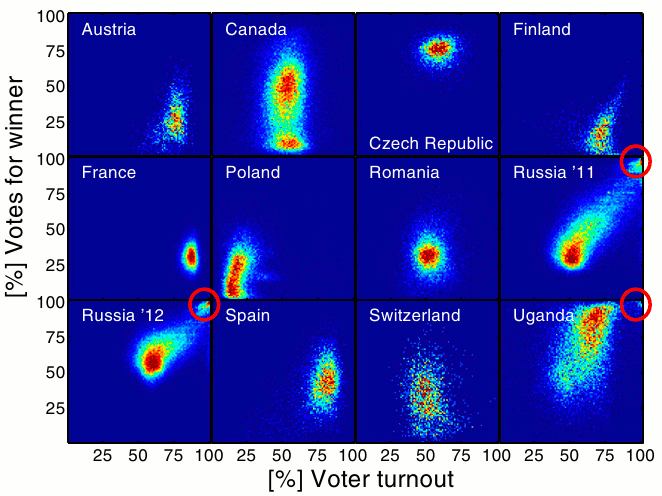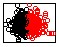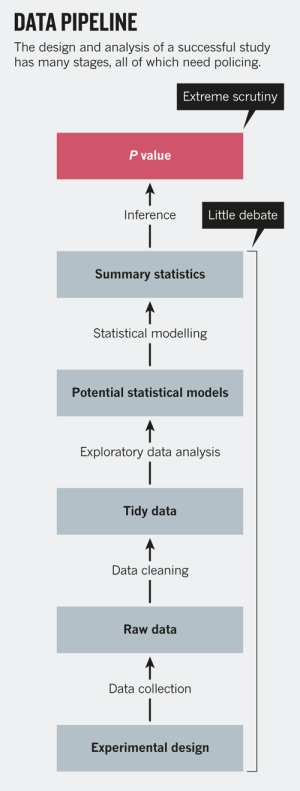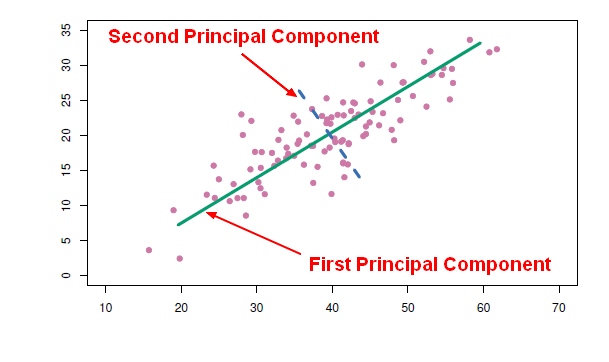# Machine Learning - Unsupervised Learning ( Mining )

Unsupervised learning is the second type of function that an algorithm can perform.

The algorithm is said to be unsupervised when no response is used in the algorithm.

Unsupervised Learning has the goal of discovering relationships and patterns rather than of determining a particular value as in supervised learning. There is:

Unsupervised learning can be used:

• for descriptive purposes.
• to find groups of samples that behave similarly,
• to find features that behave similarly,
• to find linear combinations of features with the most variation. (pca ?)
• to classify (Clustering models can be applied to classify cases according to their cluster assignments)

Unsupervised learning can be useful as a pre-processing step of supervised learning.

## Example

Example Illustrate the Model
Determine distinct segments of a population and the attribute values indicating an individual’s membership in a particular segment Clustering
Determine the five items most likely to be purchased at the same time as item X. Market Basket Analysis (or association)

Discover More(Machine|Statistical) Learning - (Target|Learned|Outcome|Dependent|Response) (Attribute|Variable) (Y|DV)

An (outcome|dependent) variable is ameasure that we want to predict. : the original score collected : the predicted score (or estimator) from the equation. The hat means “estimated” from the...(Statistics|Probability|Machine Learning|Data Mining|Data and Knowledge Discovery|Pattern Recognition|Data Science|Data Analysis)

The terms pattern recognition, machine learning, data mining and knowledge discovery in databases (KDD) are hard to separate, as they largely overlap in their scope.Machine Learninsupervised learning methodKDD...Data Mining - (Anomaly|outlier) Detection

The goal of anomaly detection is to identify unusual or suspicious cases based on deviation from the norm within data that is seemingly homogeneous. Anomaly detection is an important tool: in data...Data Mining - (Descriptive|Discovery) (Analysis|Statistics)

Descriptive analysis is also known as Descriptive statistics They are procedures used to summarize, organize, and simplify data. Descriptive function are always unsupervised See also . Visual...Data Mining - (Function|Model)

The model is the function, equation, algorithm that predicts an outcome value from one of several predictors. During the training process, the models are build. A model uses a logic and one of several...Data Mining - (Life cycle|Project|Data Pipeline)

Data mining is an experimental science. Data mining reveals correlation, not causation. With good data, you will make good algorithm. The most preferable solution is then to work on good features....Data Mining - Principal Component (Analysis|Regression) (PCA|PCR)

Principal Component Analysis (PCA) is a feature extraction method that use orthogonal linear projections to capture the underlying variance of the data. By far, the most famous dimension reduction approach...Machine Learning - (Supervised|Directed) Learning ( Training ) (Problem)

Supervised Learning has the goal of predicting a value (outcome) from particular characteristics (predictors) that describes some behaviour. The attribute used to trained and being predicted is called...Statistics - Generalized Linear Models (GLM) - Extensions of the Linear Model

The Generalized Linear Model is an extension of the linear model that allows for lots of different,non-linear models to be tested in the context of regression. GLM is the mathematical framework used in...Statistics - Sample (Variable | Attribute | Feature)

A (statistician|data miner) studying a population would be interested in collecting information different characteristics of the subject (like their length, or weight, or age) in a sample. Those characteristics...